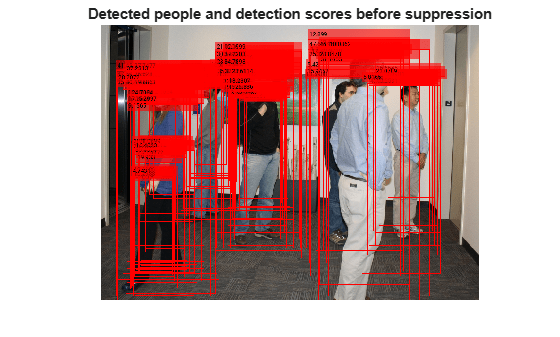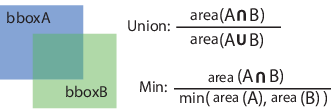# selectStrongestBbox

Select strongest bounding boxes from overlapping clusters

## Syntax

``````[selectedBbox,selectedScore] = selectStrongestBbox(bbox,score)``````
``````[selectedBbox,selectedScore,index] = selectStrongestBbox(bbox,score)``````
``````[___] = selectStrongestBbox(___,Name,Value)``````

## Description

example

``````[selectedBbox,selectedScore] = selectStrongestBbox(bbox,score)``` returns selected bounding boxes that have a high confidence score. The function uses nonmaximal suppression to eliminate overlapping bounding boxes from the `bbox` input.```
``````[selectedBbox,selectedScore,index] = selectStrongestBbox(bbox,score)``` additionally returns the `index` vector associated with `selectedBbox`. This vector contains the indices of the selected boxes in the `bbox` input.```
``````[___] = selectStrongestBbox(___,Name,Value)``` uses additional options specified by one or more `Name,Value` pair arguments.```

## Examples

collapse all

Load the pretrained aggregate channel features (ACF) people detector.

`peopleDetector = peopleDetectorACF();`

Detect people in an image. Disable the default nonmaximal suppression used by the detector.

```I = imread('visionteam1.jpg'); [bbox,score] = detect(peopleDetector,I,'SelectStrongest',false); ```

Run nonmaximal suppression with custom threshold.

```I = imread('visionteam1.jpg'); [selectedBbox,selectedScore] = selectStrongestBbox(bbox,score,'OverlapThreshold',0.3);```

Display the results.

```I1 = insertObjectAnnotation(I,'rectangle',bbox,score,'Color','r'); I2 = insertObjectAnnotation(I,'rectangle',selectedBbox,selectedScore,'Color','r'); figure, imshow(I1); title('Detected people and detection scores before suppression'); ``````figure, imshow(I2); title('Detected people and detection scores after suppression');```## Input Arguments

collapse all

Bounding boxes, specified as an M-by-4 or M-by-5 nonsparse numeric matrix. M is the number of bounding boxes. Each row of the matrix defines a bounding box as either an axis-aligned rectangle or a rotated rectangle. This table describes the format for each bounding box.

Bounding BoxRowDescription
Axis-aligned rectangle[xmin, ymin, width, height]This type of bounding box is defined in pixel coordinates as an M-by-4 matrix representing M bounding boxes
Rotated rectangle[xcenter, ycenter, width, height, yaw]This type of bounding box is defined in spatial coordinates as an M-by-5 matrix representing M bounding boxes. The xcenter and ycenter coordinates represent the center of the bounding box. The width and height elements represent the length of the box along the x and y axes, respectively. The yaw represents the rotation angle in degrees. The amount of rotation about the center of the bounding box is measured in the clockwise direction.

Data Types: `single` | `double` | `int8` | `int16` | `int32` | `uint8` | `uint16` | `uint32`

Confidence score, specified as an M-by-1 vector. The Mth score corresponds to the Mth bounding box in the `bbox` input. The `selectStrongestBbox` function uses nonmaximal suppression to eliminate overlapping bounding boxes and associate the confidence score with the boxes. A higher score represents a higher confidence in keeping the bounding box. The `score` input must be real, finite, and nonsparse.

Data Types: `single` | `double` | `int8` | `int16` | `int32` | `uint8` | `uint16` | `uint32`

### Name-Value Pair Arguments

Specify optional comma-separated pairs of `Name,Value` arguments. `Name` is the argument name and `Value` is the corresponding value. `Name` must appear inside quotes. You can specify several name and value pair arguments in any order as `Name1,Value1,...,NameN,ValueN`.

Example: `'RatioType'`,`'Union'` sets the `'RatioType'` property to `'Union'`.

Ratio type, specified as the character vector `'Union'` or `'Min'`.

• Set the ratio type to `'Union'` to compute the ratio as the area of intersection between `bboxA` and `bboxB`, divided by the area of the union of the two.

• Set the ratio type to `'Min'` to compute the ratio as the area of intersection between `bboxA` and `bboxB`, divided by the minimum area of the two bounding boxes.Data Types: `char`

Overlap ratio threshold, specified as the comma-separated pair consisting of '`OverlapThreshold`' and a scalar in the range [0 1]. When the overlap ratio is above the threshold you set, the function removes bounding boxes around the reference box. Decrease this value to reduce the number of selected bounding boxes. However, if you decrease the overlap ratio too much, you might eliminate boxes that represent objects close to each other in the image.

Data Types: `single` | `double`

## Output Arguments

collapse all

Selected bounding boxes, returned as an M-by-4 or an M-by-5 matrix. The 4-element vectors represent axis-aligned rectangles and the 5-element vectors represent rotated rectangles.

The `selectedBbox` output returns the selected bounding boxes from the `bbox` input that have the highest confidence score. The function uses nonmaximal suppression to eliminate overlapping bounding boxes.

Data Types: `single` | `double` | `int8` | `int16` | `int32` | `uint8` | `uint16` | `uint32`

Scores of selected bounding boxes, returned as an M-by-1 vector. The Mth score in the `selectedScore` output corresponds to the Mth bounding box in the `selectedBbox` output.

Data Types: `single` | `double` | `int8` | `int16` | `int32` | `uint8` | `uint16` | `uint32`

Index of selected bounding boxes, returned as an M-by-1 vector. The `index` vector contains the indices to the selected boxes in the `bbox` input.

Data Types: `double`